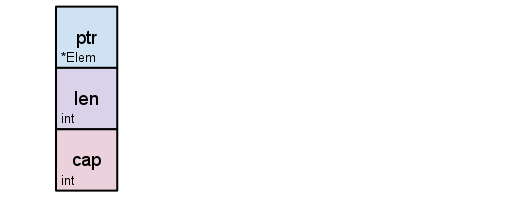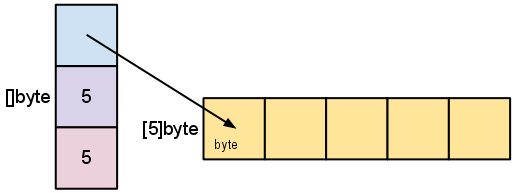## Golang: Language: Collection: Array and Slice

10th March 2021 at 8:34pm

Go 的 array 是定长的；slice 是不定长的。两者长得非常像：

• Array：类型表达为 `[N]T`，N 是长度，T 是类型，如 `int`
• Slice：类型表达为 `[]T`，T 是类型，如 `[]int`

## 构建

``````//// Array: [N]T

// l1: 声明但不赋值。此时每个元素都是 zero value
var l1 int

// l2: 声明且赋值
var l2 int = int{4, 2, 1, 3, 5}

// l3 及 l4: 自动推断类型
var l3 = int{4, 2, 1, 3, 5}
l4 := int{4, 2, 1, 3, 5}

// l5: 自动推断类型及长度（int）
l5 := [...]int{4, 2, 1, 3, 5}

//// Slice: []T
// s1: 声明但不赋值，空 slice
var s1 []int

// s2: 声明且赋值
var s2 []int = []int{4, 2, 1, 3, 5}

// s3 及 s4：自动推断类型
var s3 = []int{4, 2, 1, 3, 5}
s4 := []int{4, 2, 1, 3, 5}

// s5: 用 make() 构建
//   func make([]T, len, cap) []T
//   func make([]T, len) []T
s5 := make([]int, 5)``````

## Array 实现

Array 在 Go 的设计中是 值类型的。在 Go blog 中这么描述的：

Go's arrays are values. An array variable denotes the entire array; it is not a pointer to the first array element (as would be the case in C). This means that when you assign or pass around an array value you will make a copy of its contents. (To avoid the copy you could pass a pointer to the array, but then that's a pointer to an array, not an array.) One way to think about arrays is as a sort of struct but with indexed rather than named fields: a fixed-size composite value.

``````a := [...]int{1, 1, 1}
b := a
a = 0
fmt.Println(a, b)``````
``[0 1 1] [1 1 1]``

## Slice 实现

Slice 支持以下标索引形式读写元素值。但是对超出长度部分的写入会报 runtime error：

``````// len(b) 为 2
b := make([]int, 2, 4)
b = 1
b = 2
b = 3   // runtime error``````

Slice 的空值是 nil。`cap()` `len()` 在此情况下会返回 0。

Slice 本质上是指向某块内存区域的结构信息：Slice 支持 slicing 操作，slice 出来的结果仍然是 slice，能被读取但是无法被写入：

``````b := []int{1, 2, 3, 4}
c := b[2:]             // c: {3, 4}
b[2:] = []int{5, 6}    // compile error``````

Slicing 操作是不复制 slice 中元素的，它生成的 slice 跟原 slice 指向同块区域。因此注意 slicing 后的 len 及 cap：

``````b := make([]int, 2, 4)
b = 1
b = 2

c := b[2:]

fmt.Printf("len(b): %v, cap(b): %v\n", len(b), cap(b))
fmt.Printf("len(c): %v, cap(c): %v\n", len(c), cap(c))``````
``````len(b): 2, cap(b): 4
len(c): 0, cap(c): 2``````

• 并不是 `slice.append()`
• 而是 `slice = append(slice, V)`

``````b := make([]int, 4)
fmt.Printf("len(b): %v, cap(b): %v\n", len(b), cap(b))

b = append(b, 0)
fmt.Printf("len(b): %v, cap(b): %v\n", len(b), cap(b))``````
``````len(b): 4, cap(b): 4
len(b): 5, cap(b): 8``````

### Slice 合并

``s := append(s1, s2...)``